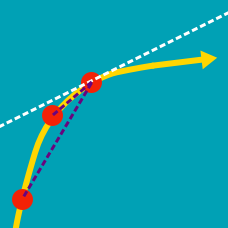Calculus

Derivatives of Logarithmic Functions

When $f(x) = \ln ( x^{13} )$, what is the value of $f'(37)?$

Details and assumptions

$\ln$ is the logarithm base $e$, also called the natural logarithm.

If $f(x) = 4 \ln (e^ {5} x)$, what is the value of $f'(1)$?

If $f(x) = \log_{2}{x}$, what is $f'(x)$?

For $y=6\ln x+9x,$ what is the value of $x \cdot \frac{dy}{dx} ?$

If $f(x) = \log_{3}{(x+2)}$, what is $f'(x)$?

×